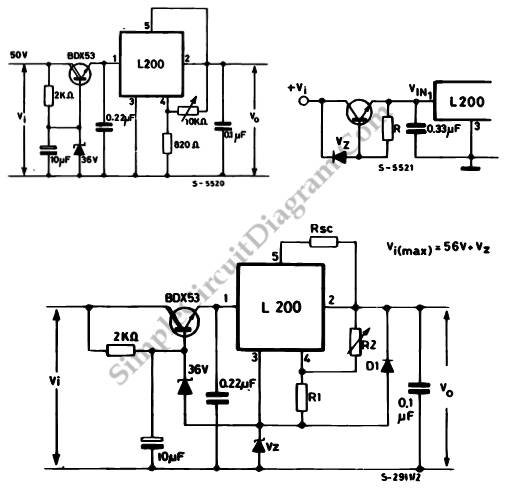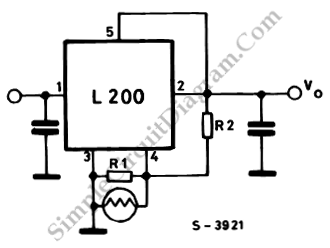## Wideband RF AmplifierThis is a circuit of Wideband RF amplifiers. This circuit use current-feedback components like the THS3202. The THS3202 was chosen because it has fast slew rate and wide bandwidth. The amplifier voltage gain of this circuit is 20 and stage voltage gain of this circuit is 10. Here is the circuit: Compared to traditional RF circuitry, this circuit is simpler. […]

## Higher Input Or Output for L200 Voltage RegulatorThis is higher input or output for L200 voltage regulator circuit. This circuit is designed to solve the problem that occurs when the input or output voltages are higher than the device can produce. This circuit uses the external components. Here is the circuit : The transistor is used to absorb the excess voltage when there are high input voltage. […]

## L200 Light ControllerThis is a circuit of L200 light controller. The output of this circuit is controlled by the surrounding environment’s brightness.  If the brightness increases, the output voltage will increases as well. Here is the circuit : The R1 and photoresistor are connected in parallel. They are used as regulator.  If we connect the the photoresistor in parallel with R2, we […]